# Backpropagation of Machine Learning

Last updated on：2 years ago

Backpropagation is a rather basic concept of machine learning. I want to write a concise review here.

# Classes review version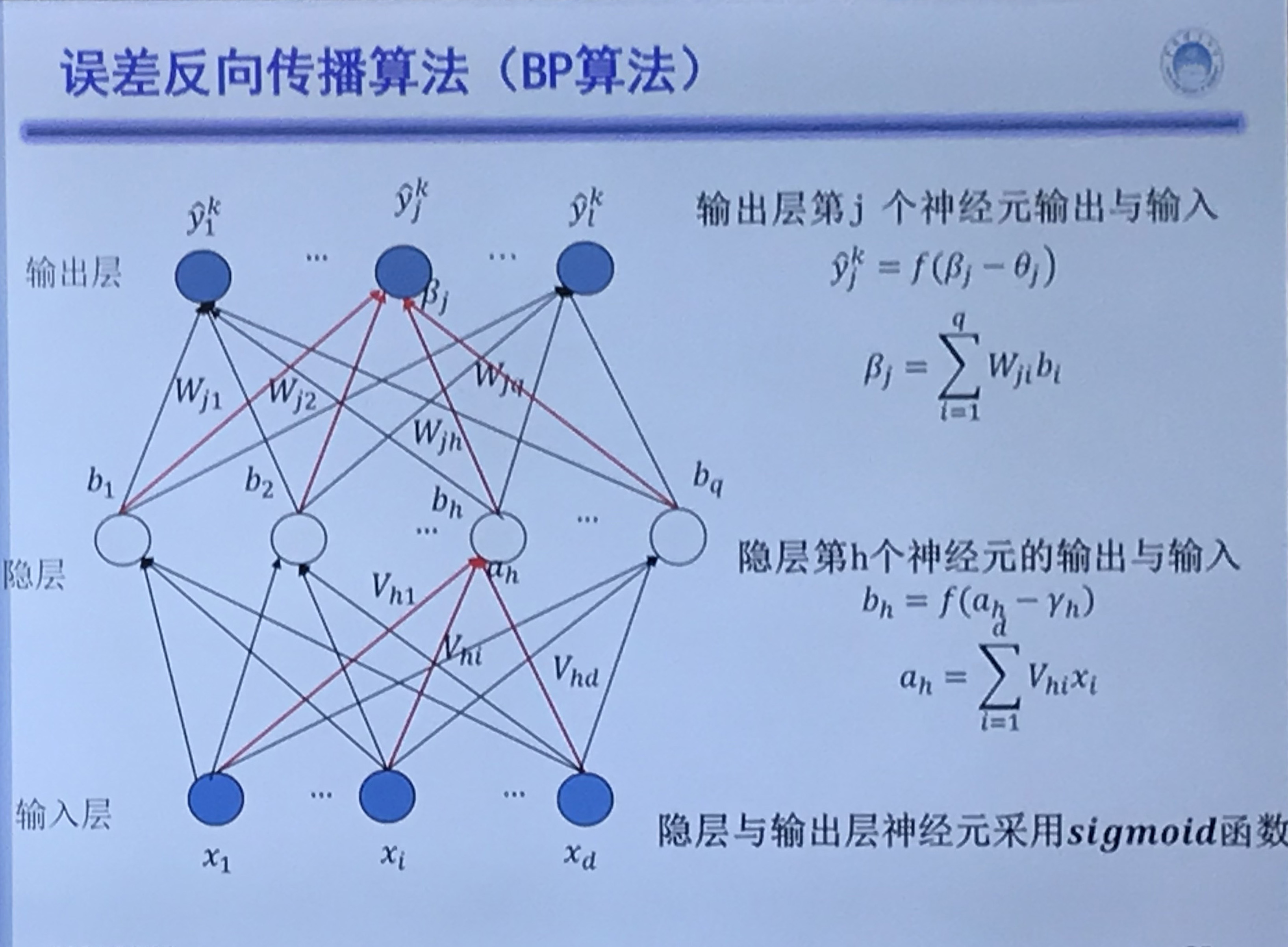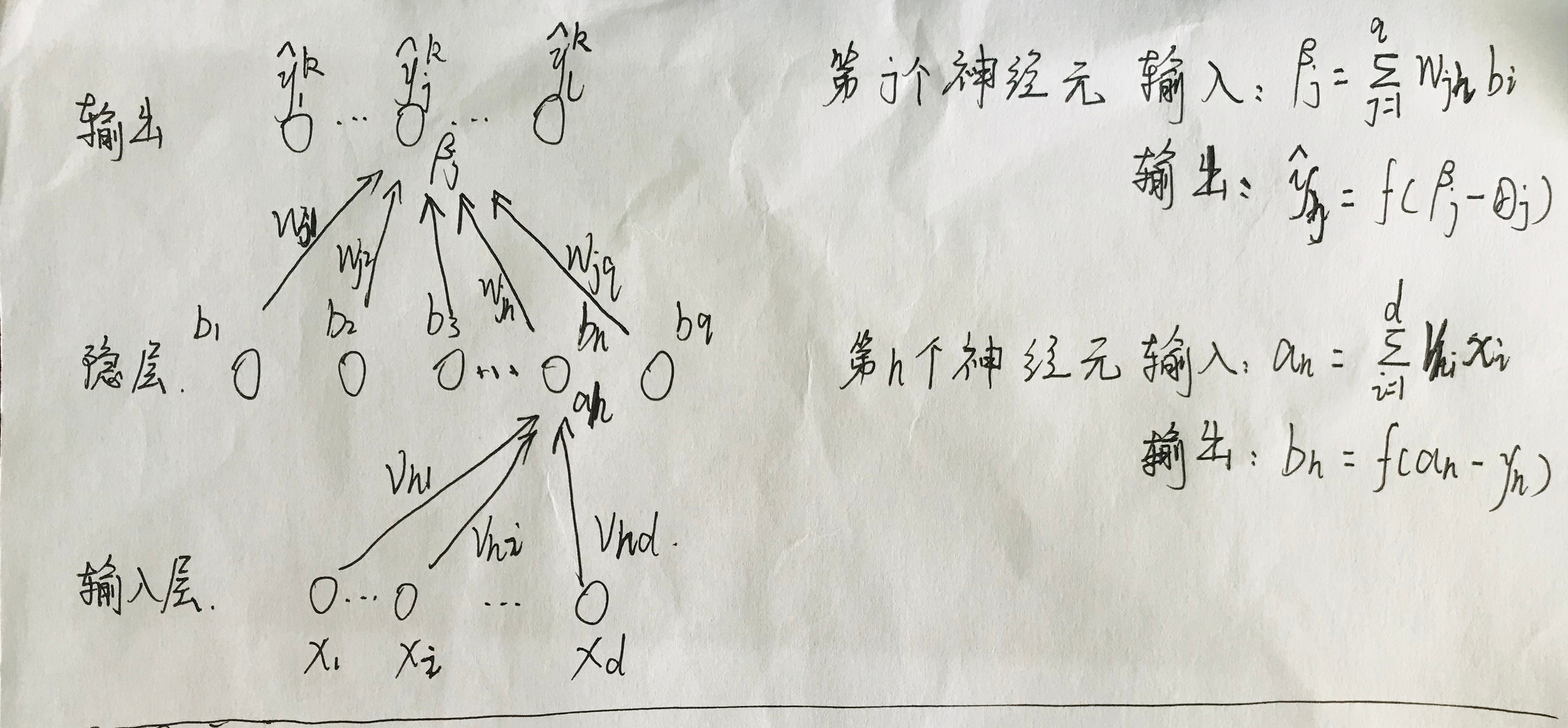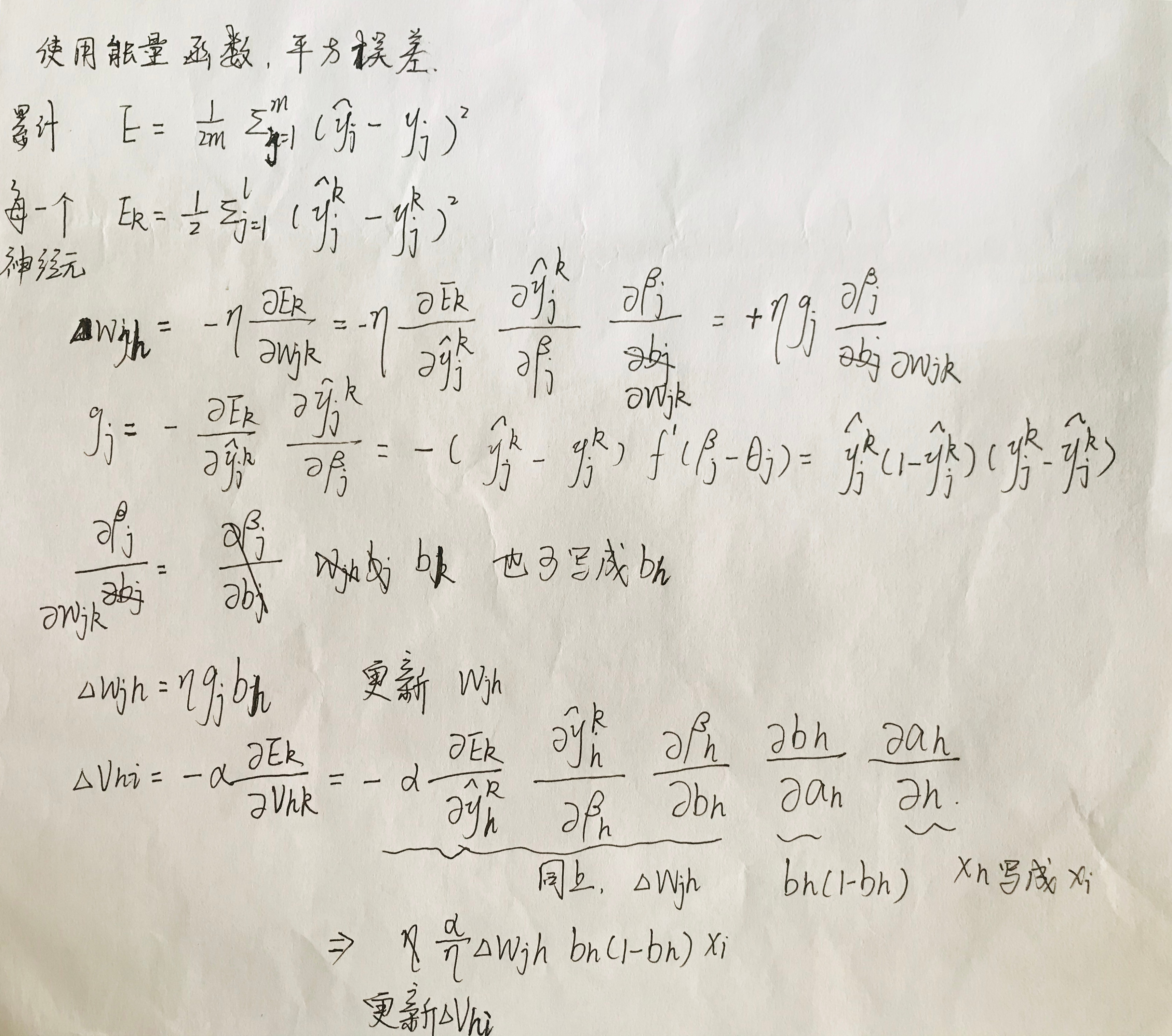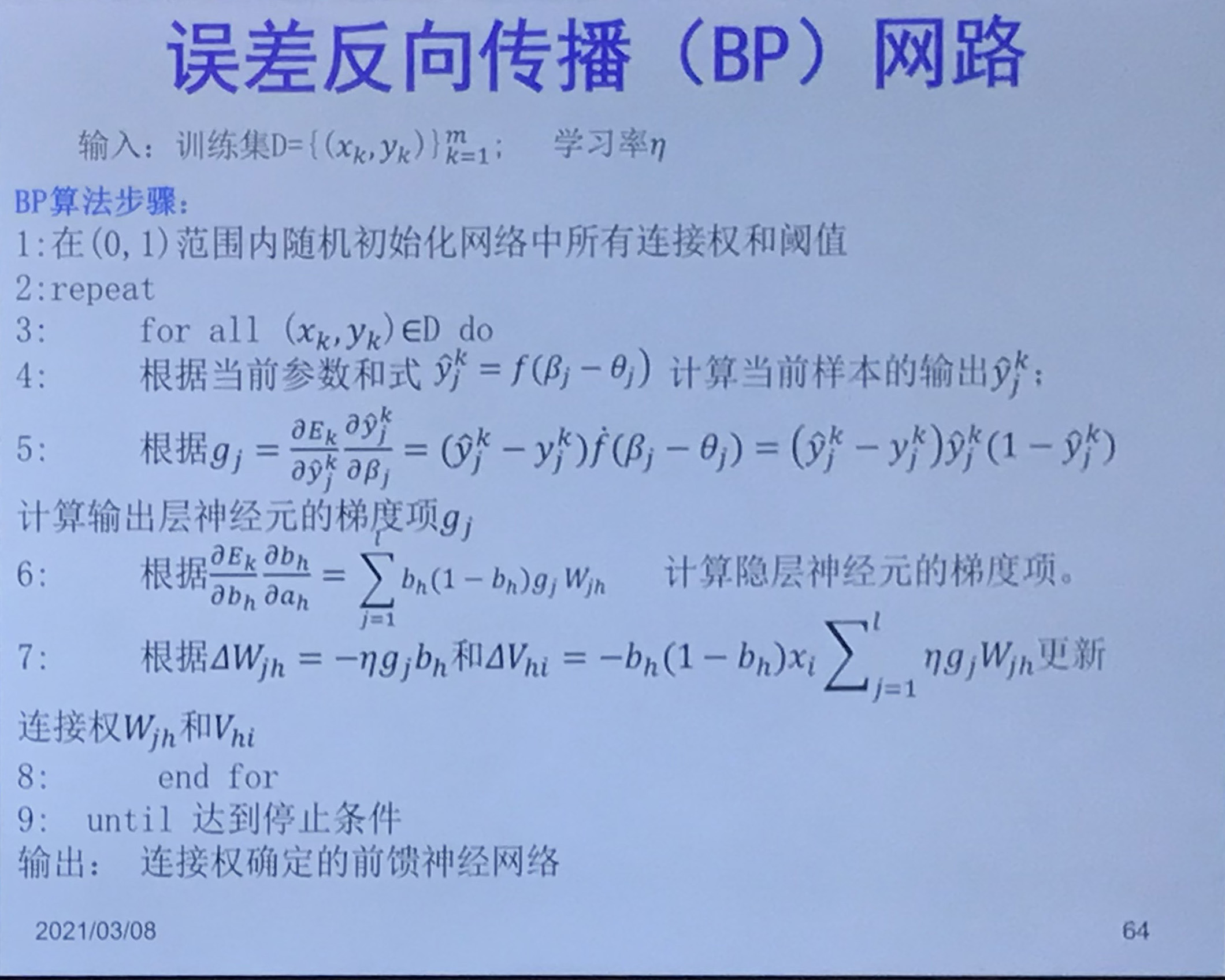# Andrew NG

$a^{(i)}$ is output, $z^{(i)}\ \text{or}\ x$ is input, $\theta ^{( i )}$ is weight, $g(x)$ is activation function.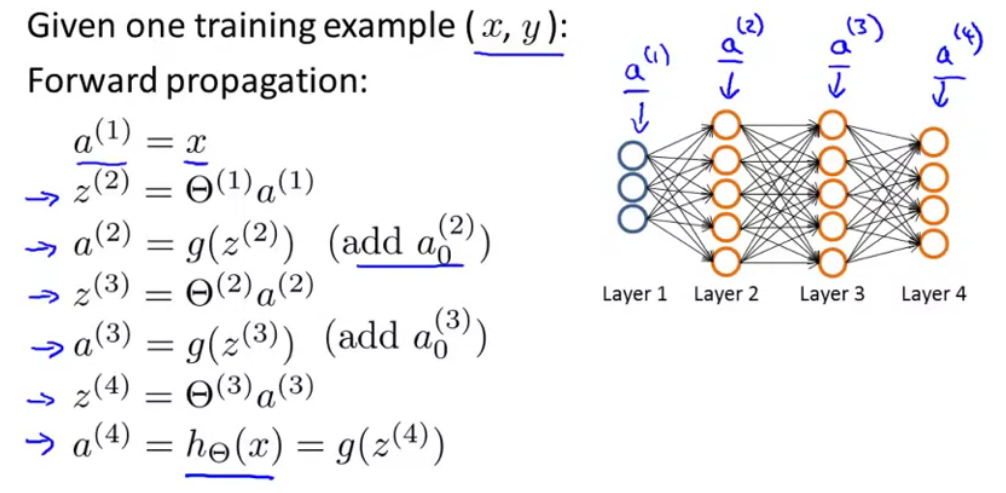Compute the delta terms for the earlier layer.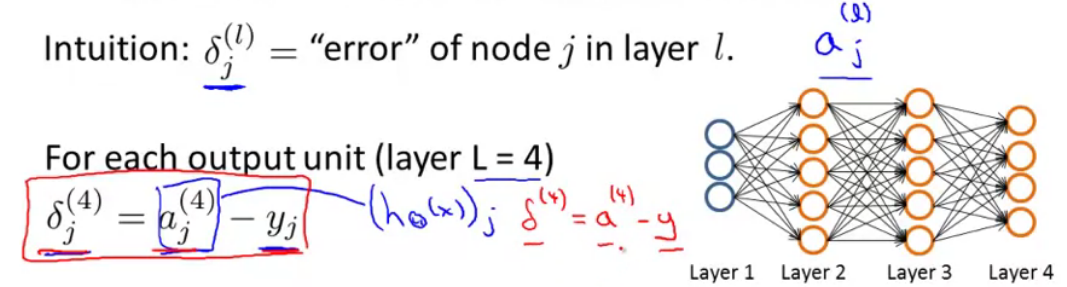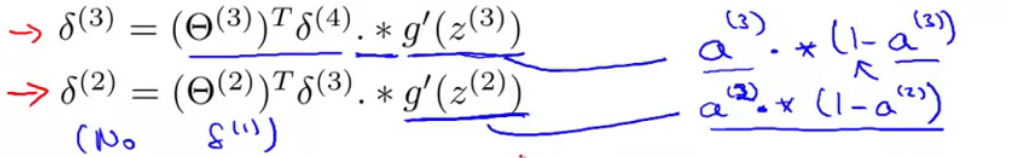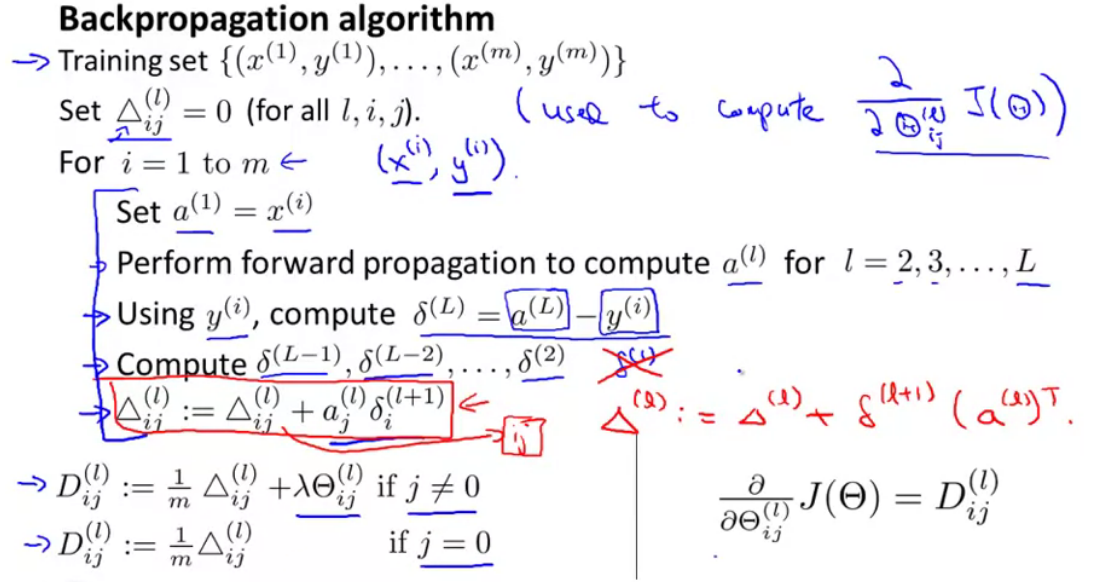# Training a neural network steps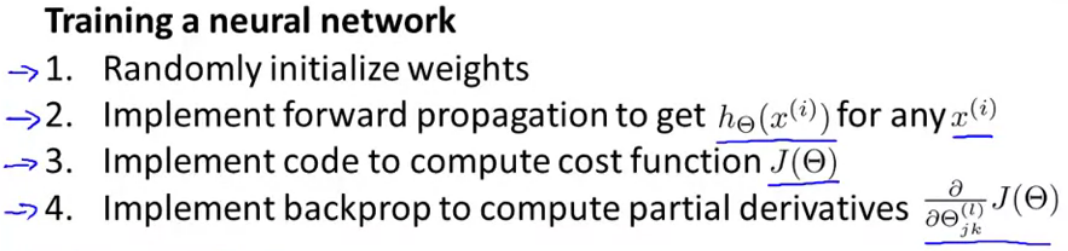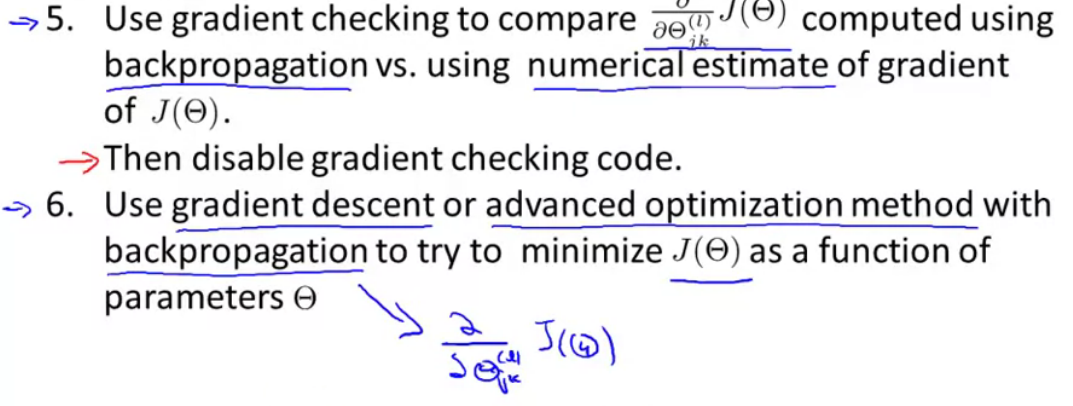# Reference

 Andrew NG, Machine learning